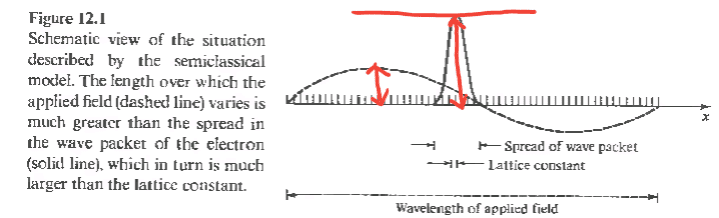# Semiclassical model of electron dynamics

• I
Summary:
assumptions of semiclassical model of electron dynamics
Why do we exclude interband transitions in semiclassical model of electton dynamics? I mean why it is an assumption in this model?

Lord Jestocost
Gold Member
Let me quote a section from Evgeny Tsymbal's lecture "Electron Dynamics and Fermi Surfaces":

"The next important subject we address is electron dynamics in metals. Our consideration will be based on a semiclassical model. The term “semiclassical” comes from the fact that within this model the electronic structure is described quantum-mechanically but electron dynamics itself is considered in a classical way, i.e. using classical equations of motion. Within the semiclassical model we assume that we know the electronic structure of metal, which determines the energy band as a function of the wave vector. The aim of the model is to relate the band structure to the transport properties as a response to the applied electric field.
Given the functions ##E_n(k)## the semiclassical model associates with each electron a position, a wave vector and a band index ##n##. In the presence of applied fields the position, the wave vector, and the index are taken to evolve according to the following rules:
(1) The band index is a constant of the motion. The semiclassical model ignores the possibility of interband transitions. This implies that within this model it assumed that the applied electric field is small......"

https://unlcms.unl.edu/cas/physics/tsymbal/teaching/SSP-927/index.shtml (see lecture note 10. Electron Dynamics and Fermi Surfaces)

•Rzbs
Thanks but I can't understand why in this model we need to consider applied electric field small? I only know it must be small in comparison to amplitude of wave packet as shown in the following picture, but I don't know why (this picture is for showing wave vectors but it also shows amplitudes):Last edited:
Lord Jestocost
Gold Member
Let me quote Neil W. Ashcroft and N. David Mermin (from „Solid State Physics“, Chapter 12):

"In the limit of zero periodic potential the semiclassical model must break down, for in that limit the electron will be a free electron. In a uniform electrical field a free electron can continually increase its kinetic energy at the expense of electrostatic potential energy. However, the semiclassical model forbids interband transitions, and therefore requires that the energy of any electron remains confined within the limits of the band in which the electron originally found itself."

I know these things but I don't know why I can't relate these two facts to each other: small applied electric field and exclude interband transitions.

Can you please explain what happens in this model if the applied electric field wasn't small?

Thanks

Lord Jestocost
Gold Member
The semiclassical model of the electron dynamics in crystals is based upon the assumption that the wave packet representing the electron is a superposition of Bloch states from a single band. That’s the approximation. In case large electric fields are applied to a crystal, the probability for electron transitions into other bands under the action of the electric field (owing to interband tunneling (Zener effect)) can no longer be neglected. By construction, the semiclassical model cannot account for such effects. Its validity is thus restricted to a certain range regarding the electric field strength.

•Rzbs
The semiclassical model of the electron dynamics in crystals is based upon the assumption that the wave packet representing the electron is a superposition of Bloch states from a single band
This is the thing I didn't consider and now help me to understand...
•